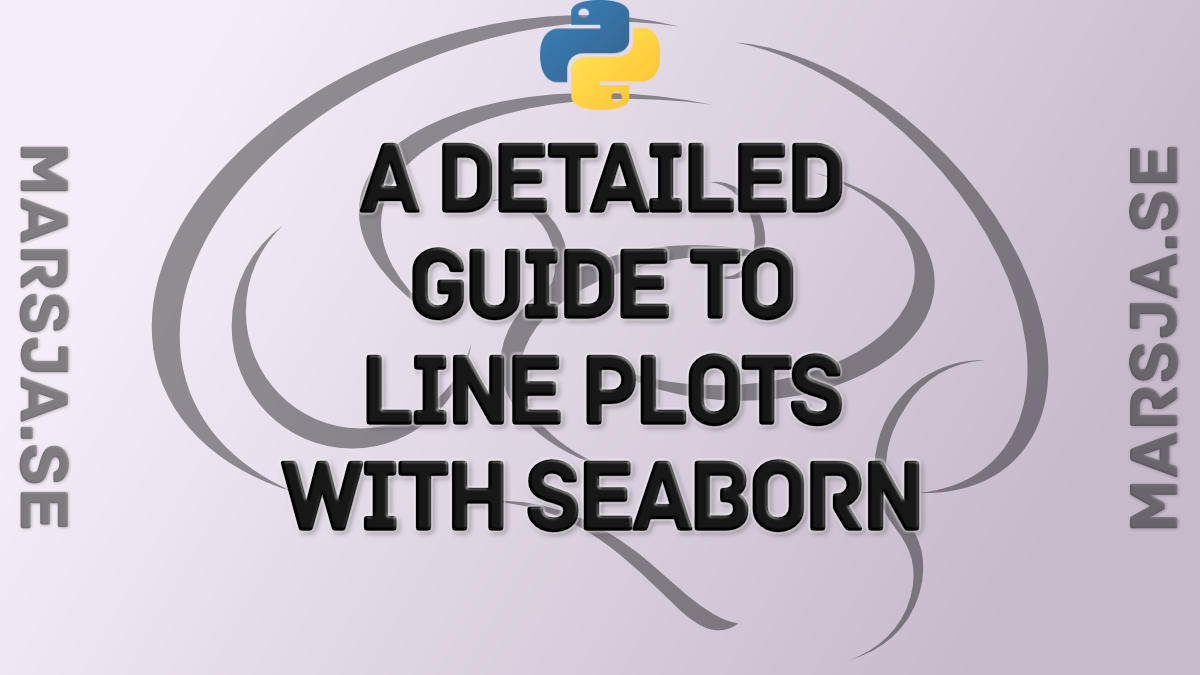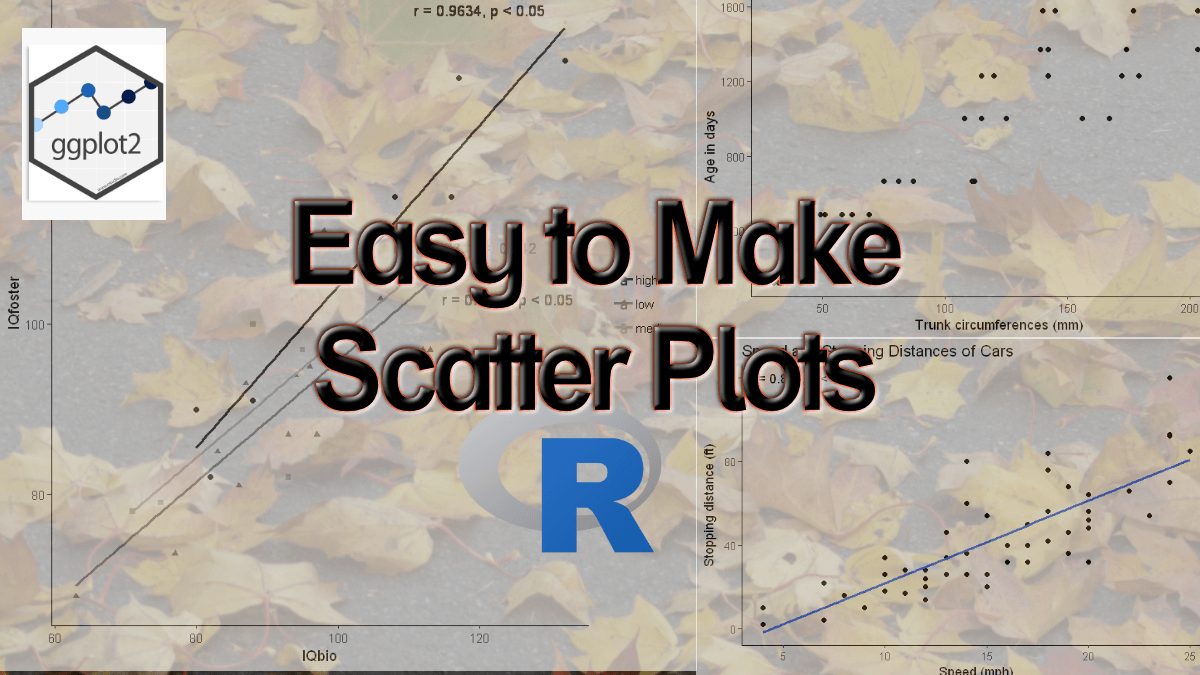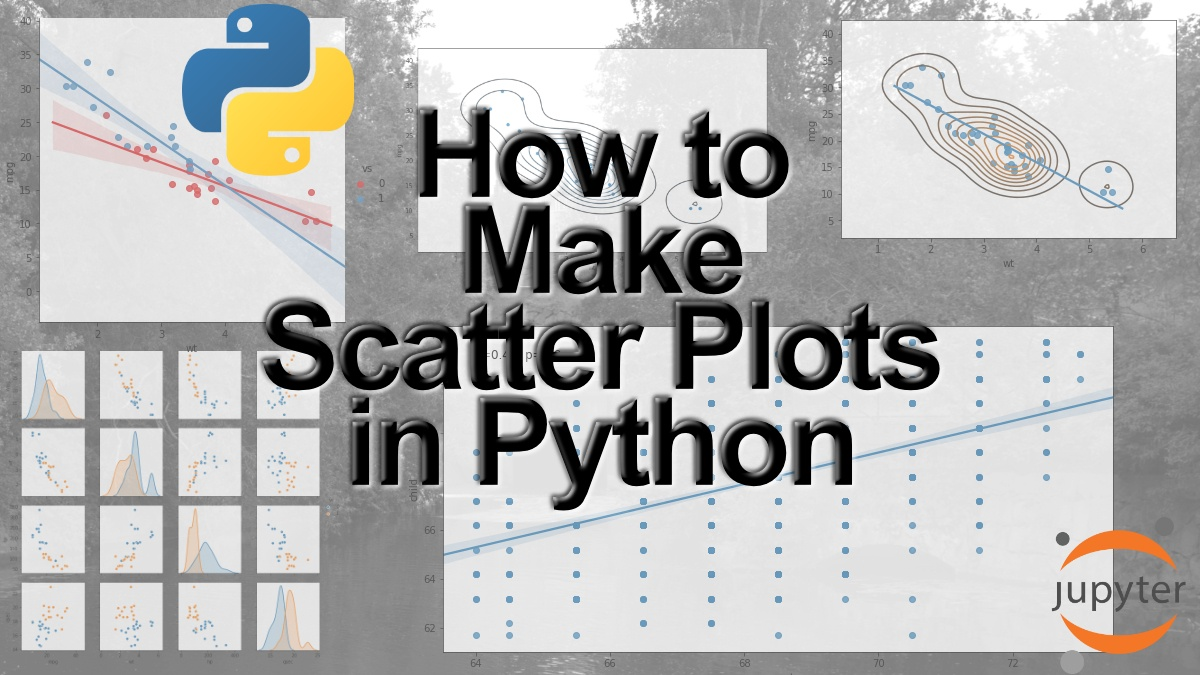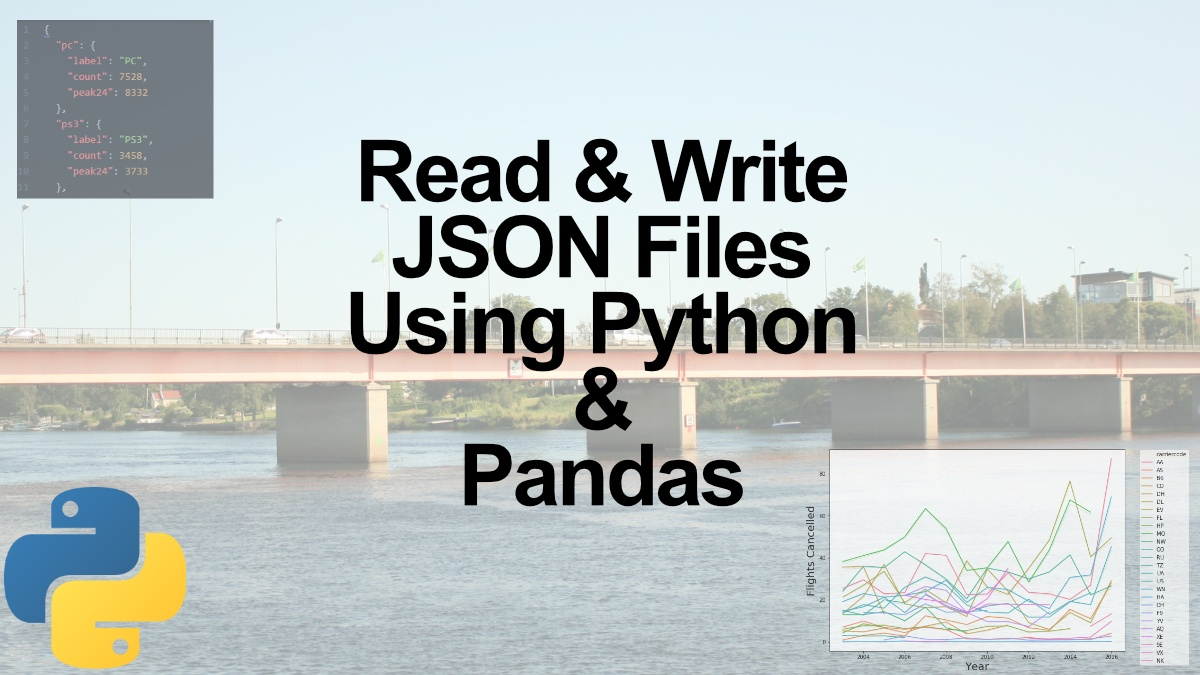## Seaborn Line Plots: A Detailed Guide with Examples (Multiple Lines)

In this Python data visualization tutorial, we will learn how to create line plots with Seaborn. First, we’ll start with the simplest example (with one line) and then we’ll look at how to change the look of the graphs, and how to plot multiple lines, among...## How to Change the Size of Seaborn Plots

In this short tutorial, we will learn how to change the figure size of Seaborn plots. For many reasons, we may need to either increase the size or decrease the size, of our plots created with Seaborn. How to Make Seaborn Plot Bigger Now, if we only want to know how to...## How to Make a Scatter Plot in R with Ggplot2

In this post, we will learn how make scatter plots using R and the package ggplot2. More specifically, we will learn how to make scatter plots, change the size of the dots, change the markers, the colors, and change the number of ticks.  Furthermore, we will...## How to Make a Scatter Plot in Python using Seaborn

Data visualization is a big part of the process of data analysis. In this post, we will learn how to make a scatter plot using Python and the package Seaborn. In detail, we will learn how to use the Seaborn methods scatterplot, regplot, lmplot, and pairplot to create...## How to Read and Write JSON Files using Python and Pandas

In this post, we will learn how to read and write JSON files using Python. In the first part, we are going to use the Python package json to create and read a JSON file as well as write a JSON file. After that, we are going to use Pandas read_json method to load JSON...